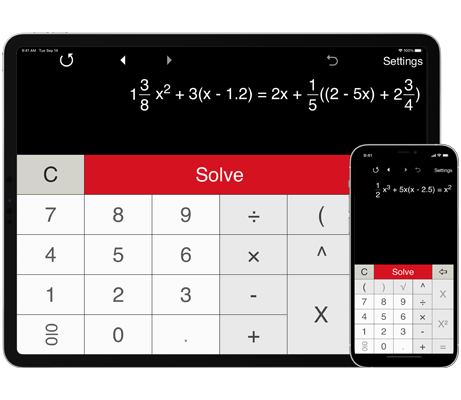# Linear equation solver

Solves a wide range of linear equations in one variable.
Solves linear equations with integers, fractions and decimals.
Solves linear equations with brackets.
Shows calculation details.

## Solving first degree equations step by step

Linear Equation Solver solves a wide range of linear equations (first degree equations) in one variable. Just enter a linear equation and click 'Solve'.

Features:

Solving one-step linear equations.

Solving two-step linear equations.

Solving multi-step linear equations.

Linear Equation Solver keeps history with the possibility to recall previous equations.

Linear Equation Solving Calculator provides built-in templates for solving first degree equations in the forms ax + b = c and ax + b = cx + d.

Note: Linear Equation Solver does not solve first degree equations with a variable in the denominator.